Thread: Eric Dollard View Single Postt-rexSenior Member Join Date: Aug 2011 Location: In the bushes Posts: 422
The Law of E.M. Induction, Part 6 (3 of 3)

(8) In the employment of parameter variation for the development of an E.M.F., this thru variation of the current, the objective is to develop a back E.M.F. of self induction. The E.M.F. facilitates the flow of energy but this flow is wattless. Here the reactance coil serves as a dissapationless resistor for A.C. circuits. All energy is conserved, what goes into the magnetism all comes back out of the magnetism. The Law of Energy Continuity is not a consideration here. The alternative condition is the development of an electro-motive force by the variation of the inductance. This E.M.F. represents the transfer of energy into, or out of, the magnetism. However the energy is not conserved, thus the Law of Energy Continuity must be applied to identify the location of the transferred energy, (motion, heat, aether, etc).

(9) Parameter Variation of the inductance is expressed by the relation,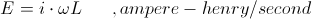And here it is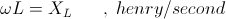In comparison, for the condition of parameter variation of the current the relation is,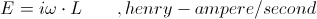And here it is given,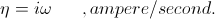Hence,

Current variation in ampere per second,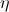, and inductance variation in henry per second, XL, two distinct variations.

In these two distinctly different conditions, each have the same component dimensions,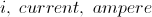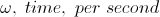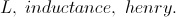These three dimensional relations engender but one magnetic field. However the order in which these three dimensions are situated gives rise to two distinctly different conditions, that is,

Ampere  Henry per Second,

Henry  Ampere per Second.

The Law of E.M. Induction exists in two forms with the same basic dimensions. Hence the term reactance can take on two new meanings, the reactance of self inductance, and the reactance of inductance variation,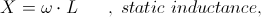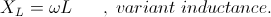Only in the variant inductance are the dimensions correctly in the henry per second.

(10) For the condition of current variation with respect to time the inductance is a constant, or invariant. The alternating E.M.F. is produced by a current alternating between maximum values of opposite polarity, positive and negative. A zero crossing results, between maximum values.

For the condition of inductance variation with respect to time the current can be constant, a direct current, that is, invariant. The alternaing E.M.F. is produced by the pulsation of inductance between maximum and minimum values. There is no zero inductance. Both the maximum and minimum values are positive.

Where it is possible to reverse the current, or make it pulsating, the inductance cannot be reversed. Negative inductance is undefined. The inductance variation is intrinsically asymmetrical in nature. The variation exists as a percentage of modulation, 100 percent being variation of inductance between zero inductance, and the maximum inductance, values, as limits.

(11) This parameter variation can be extended to both the current and the inductance, both in variation together. Each can be in variation at their own time rates, each at its own frequency and phase angle. This gives rise to a complex E.M.F. containing modulation products of sum and difference frequencies, with the originating frequencies suppressed. This is known as a double sideband modulation. The E.M.F. is of the form,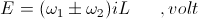Here enters chapter 25, of Steinmetz's A.C. book, 1916 edition. The chapter is titled Distortion of Waveshape and Its Cause. Here Steinmetz develops expressions for what is called Synchronous Parameter Variation, for both rotating and static apparatus. This serves as an extension on the Theory and Laws of Hysteresis.

BK DE N6KPH
__________________
SUPPORT ERIC DOLLARD'S WORK AT EPD LABORATORIES, INC.

Purchase Eric Dollard's Books & Videos - 70% of the sale goes to Eric and EPD Laboratories: Eric Dollard Books & Videos
Donate by Paypal: Donate to EPD Laboratories

Last edited by t-rex; 07-25-2012 at 02:57 AM.